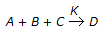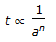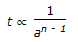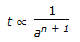# Chemical Engineering - Chemical Reaction Engineering

### Exercise :: Chemical Reaction Engineering - Section 9

21.

What is the order of chemical reaction, if itis found that the reaction rate doubles on doubling the concentration of B and also the reaction rate doubles when the concentrations of both A & B were doubled and quandrupled when the concentrations of both B & C were doubled ?

 A. 1 B. 2 C. 3 D. 4

Explanation:

No answer description available for this question. Let us discuss.

22.

Reactions with high activation energy are

 A. very temperature sensitive B. temperature insensitive C. always irreversible D. always reversible

Explanation:

No answer description available for this question. Let us discuss.

23.

If n = overall order of a chemical reaction. a = initial concentration of reactant. t = time required to complete a definite fraction of the reaction. Then pick out the correct relationship.

 A.B.C.D. t ∝ an

Explanation:

No answer description available for this question. Let us discuss.

24.

In case of a/an __________ chemical reaction, conversion increases with the rise in temperature.

 A. reversible exothermic B. irreversible exothermic C. irreversible endothermic D. reversible endothermic

Explanation:

No answer description available for this question. Let us discuss.

25.

A space velocity of 5 hr-1 means that

 A. five reactor volumes of feed (at specified conditions) are being fed into the reactor per hour. B. after every 5 hours, reactor is being filled with the feed. C. cent per cent conversion can be achieved in at least 5 hours. D. a fixed conversion of a given batch of feed takes 5 hours.